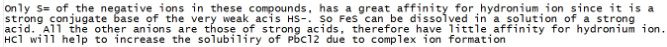# Which of the following compounds will be more soluble in acidic solution than in pure water?

Which of the following compounds will be more soluble in acidic solution than in pure water?

1. PbCl2
2. FeS
3. Ca(ClO4)2
4. CuI

METHOD:

To determine the solubility in Acidic Solution for PbCl2 in variable form. No Ksp given. ASSUME: All the above are bases.

1. PbCl2–>Pb+2 + 2Cl- (generally show the dissociation of PbCl2 in an acid solution that’s capable)
use this 2Cl- since this is essentially the Anion(electron being donated by the base PbCl2 by Lewis Acid rule)
2. 2Cl- + H+ --> HCl (use the H+ because this indicative of an acidic solution, if basic soln use OH-)
3. ICE Table in Variable Form
4. Find solubility product constant=ksp, then find actual values of ksp in text/online
5. Find Solubility of the compounds: s

WORK:

PbCl2: ksp=4s^3.
Looked in textbook ksp for PbCl2 to be 1.1710^-5
solve for s ====> s=[solubility of PbCl2]= 3.42
10^-3

FeS: ksp=s^2
where ksp= 3.7210-19
==> s=[Solubility of FeS] = 4.53
10^-7

CuI: ksp= s^2
where ksp = 1.2710^-12
s=[solubility of CuI] = 1.13
10^-6

SUMMARY:

Solubilities: [PbCl2]= 3.4210^-3 [FeS]=4.5310^-7 [CuI]=1.13*10^-6 (only did these three)
Most soluble would the be PbCl2. Correct? Is this a possible method?